Contents >> Applied Mathematics >> Matrix Algebra >> Principles of Matrix Calculation >> Powers of matrices

 Matrix Algebra - Powers of matrices Powers of matrices Let A – a square matrix and n – a natural number. Then the n -th power of the matrix A is: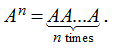Besides it is considered, that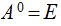, where Е – an identity matrix. If A is a regular (non-singular) matrix, it is possible to enter a negative power of a matrix: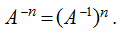For powers of matrices with the whole indices the following rules take place: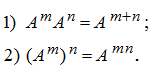If A and B – square matrices of the same orders, and АВ = ВА , then Newton’s binomial formula takes place: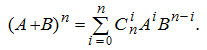E x a m p l e   .  Find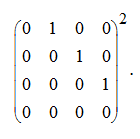S o l u t i o n .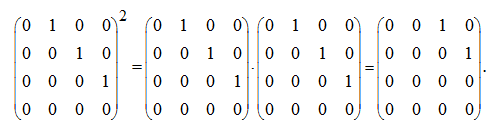< Previous Contents Next >